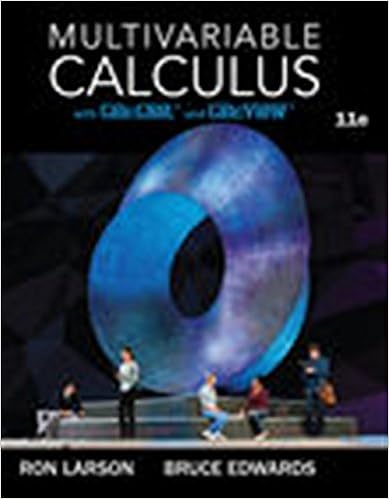# Andy_Nguyen_Math260 W5 lab - MATH260Week 5 Lab Name Andy...

• Lab Report
• 7
• 80% (5) 4 out of 5 people found this document helpful

This preview shows page 1 - 2 out of 7 pages.

##### We have textbook solutions for you!
The document you are viewing contains questions related to this textbook.The document you are viewing contains questions related to this textbook.
Chapter 13 / Exercise 36
Multivariable Calculus
LarsonExpert Verified
MATH260—Week 5 LabName: Andy NguyenCategory 1: Derivatives of Exponentials: eDirections:Look at the examples below and answer the question.f(x) = exf (x) = ef(x) = e5xf (x) = 5e5xf(x)=4ex2f '(x)=8x ex2f(x)=ex32xf '(x)=(3x22)ex32x1) Describe in your own words what to do to find the derivative for problems like those above. ux
2) Describe in your own words what to do to find the derivative for problems like those above
3) Find the derivative; show all work: f(x) = 5
##### We have textbook solutions for you!
The document you are viewing contains questions related to this textbook.The document you are viewing contains questions related to this textbook.
Chapter 13 / Exercise 36
Multivariable Calculus
LarsonExpert Verified
•••